TripleGems

# Lewis Structure of NH3 (Ammonia) (With 6 Simple Steps)Would you like to learn how to draw the Lewis structure of NH3?

The following six steps will show you how to draw the Lewis dot structure of NH3 (with images).

It’s time to start the 6 simple steps! Let’s get started!

Lewis structure of NH3 (ammonia) contains three single bonds between the Nitrogen (N) atom and each Hydrogen (H) atom. The Nitrogen atom (N) is at the center and it is surrounded by 3 hydrogen atoms (H). The Nitrogen atom has one lone pair.

The Lewis dot structure can be explained step by step if we draw it.

## The Lewis Structure of NH3 is drawn in 6 steps

Here are the 6 simple steps to draw the Lewis Structure of NH3

### 1. Determine how many electrons there are in each valence shell

A molecule of NH3 (ammonia) is given here. It is first necessary to find the number of valence electrons in the NH3 molecule in order to draw its Lewis structure. Atoms with valence electrons have electrons in their outermost shell.

Let’s start by calculating this.

NH3 valence electron calculation:

For Nitrogen:On the periodic table, nitrogen is a group of 15 elements.
Therefore, nitrogen has 5 valence electrons (see image below).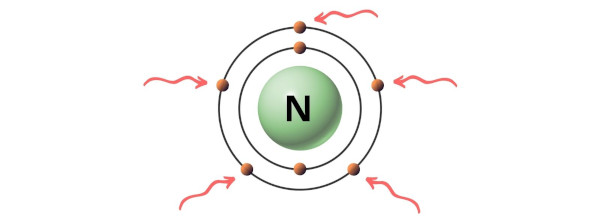• For Hydrogen:
On the periodic table, hydrogen belongs to group 1.
As a result, hydrogen has a valence electron of 1 (see below image).Therefore, in an NH3 molecule, An atom of Nitrogen (N) has 5 valence electrons. Hydrogen (H) atoms give one valence electron

NH3 contains 5 Valence electrons and 1(3) Valence electrons for a total of 8 Valence electrons.

### 2. Choose the center atom (H is always outside)

Put the least electronegative atom at the center when selecting the center atom.

In the periodic table, fluorine is the most electronegative element, and its electronegativity decreases as we move from right to left and from top to bottom.

A hydrogen atom is less electronegative than a nitrogen atom (N) in the NH3 molecule. However, hydrogen must be kept outside according to the rule.
Therefore, nitrogen should be placed in the center and the remaining three hydrogen atoms should surround it.### 3. Symbolize a chemical bond with two electrons

To represent the chemical bond between nitrogen atoms and hydrogen atoms in the above sketch, place two electrons (e.g. electron pair) between them.

In an NH3 molecule, the pairs of electrons between Nitrogen (N) and Hydrogen (H) atoms form a chemical bond, which bonds them to each other.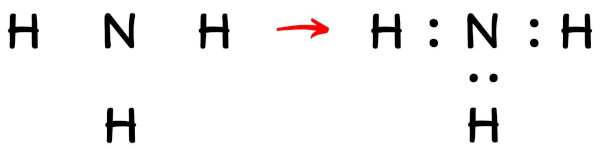### 4. Completing an octet (or duplet) on an outside atom. On the central atom, place the pair of valence electrons that are left

There’s nothing to worry about, I’ll explain! NH3’s Lewis structure has hydrogen atoms at its outer edges.

This means that you must verify whether or not these hydrogen atoms are forming a duplet! It only takes two electrons for hydrogen to have a complete outer shell.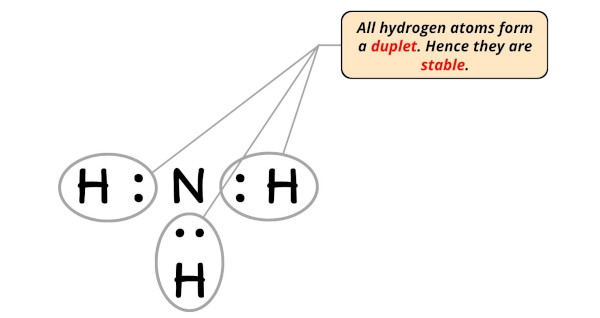All the hydrogen atoms in the above image form a duplet. Additionally, the above structure uses only six valence electrons of the NH3 molecule. As calculated in step #1, there are 8 valence electrons in the NH3 molecules.

In other words, the central atom must keep 2 electrons instead of 8 – 6.
On the central atom, let’s keep these two electrons (i.e. electron pair).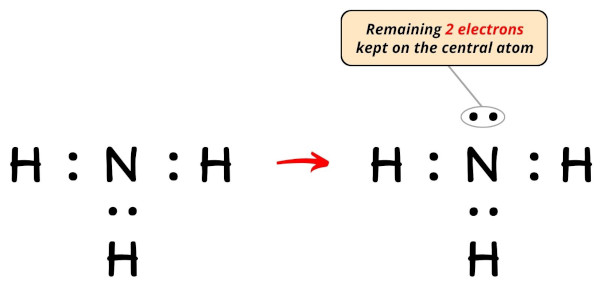### 5. Make sure the central atom has an octet. A double or triple bond is formed if it does not have an octet

During this step, we must determine whether the central atom (nitrogen) has an octet.

To put it simply, we need to check whether the central Nitrogen (N) atom has 8 electrons.This image shows that the central atom (nitrogen) has 8 electrons. The nitrogen atom is stable since it fulfills the octet rule.

### 6. Calculate the formal charge on each atom to check the stability of the Lewis structure

In the final step, you must check the formal charge of each nitrogen atom (N), as well as each hydrogen atom (H).

Formal charges can be calculated using the formula below:

Formal charge = Valence electrons – Nonbonding electrons – (Bonding electrons)/2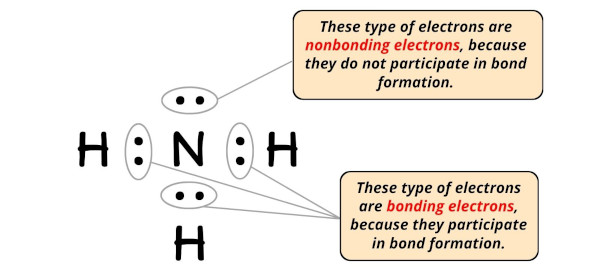• For Nitrogen:
Valence electrons = 5 (as it is in group 15)
Nonbonding electrons = 2
Bonding electrons = 6
• For Hydrogen:
Valence electron = 1 (as it is in group 1)
Nonbonding electrons = 0
Bonding electrons = 2

As you can see above, nitrogen and hydrogen have “zero” formal charges.
Thus, the above Lewis structure of NH3 will not change, and it is the only final stable structure.

NH3’s Lewis dot structure represents single bonds as electron pairs (:).